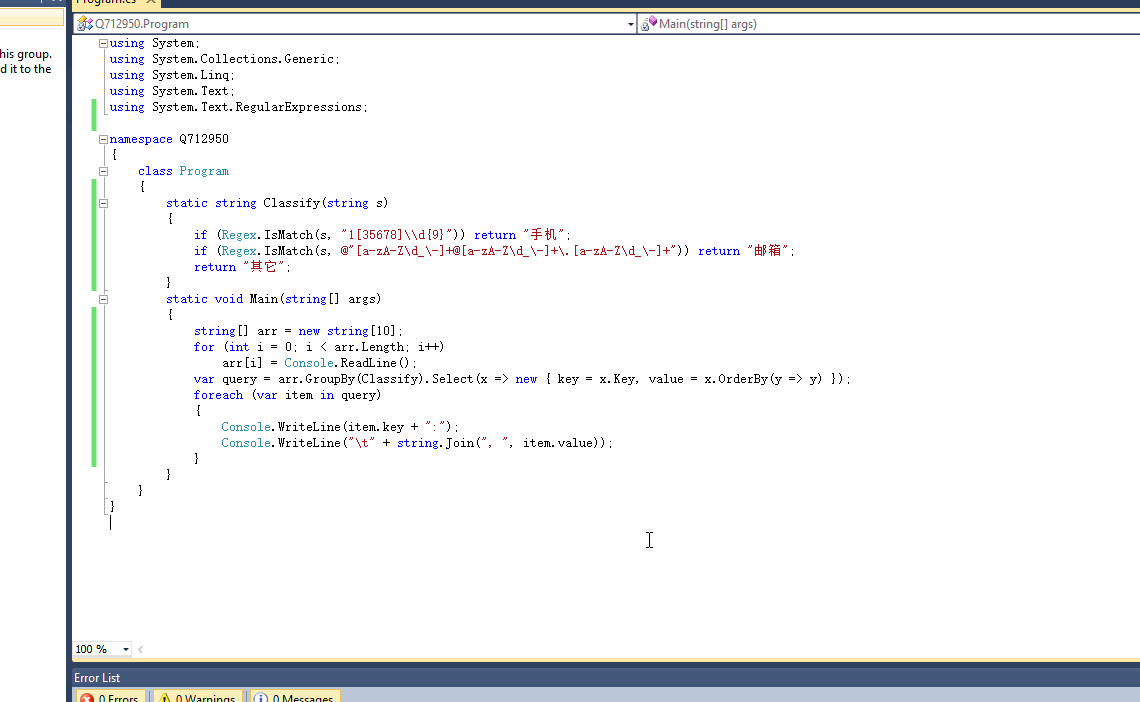c# 在命令行中输入任意10个字符串，根据字符串类型将输入的数据放入到不同数组中，最后将数组进行排序

1、输入的字符串，可以是手机、邮箱、身份证等
2、只将手机、邮箱的字符串筛选出来放到两个数组中，其余的放入yi'ge'shu'zu'zhon
3、数组按照升序排序，比如数组[6,3,8,2,4]，按照升序排序后结果为[2,3,4,6,8]

1个回答

``````using System;
using System.Collections.Generic;
using System.Linq;
using System.Text;
using System.Text.RegularExpressions;

namespace Q712950
{
class Program
{
static string Classify(string s)
{
if (Regex.IsMatch(s, "1\\d{9}")) return "手机";
if (Regex.IsMatch(s, @"[a-zA-Z\d_\-]+@[a-zA-Z\d_\-]+\.[a-zA-Z\d_\-]+")) return "邮箱";
return "其它";
}
static void Main(string[] args)
{
string[] arr = new string;
for (int i = 0; i < arr.Length; i++)
var query = arr.GroupBy(Classify).Select(x => new { key = x.Key, value = x.OrderBy(y => y) });
foreach (var item in query)
{
Console.WriteLine(item.key + ":");
Console.WriteLine("\t" + string.Join(", ", item.value));
}
}
}
}

```````如果问题得到解决，请点我回答左上角的采纳，谢谢`*题目要求* **Problem Description 从键盘输入任意一个字符串和一个字符，要求从该字符串中删除所有该字符。 Input 输入有多组测试数据。 每组两行，第一行是字符串（字符串至少还有一个字符，不多于100个），第二行是一个字符 Output 每组输出一行，删除了所有应删除字符后的字符串 Sample Input ABCDE E ASD Dfg fhd D Sample Output ABCD AS fg fhd** *我的代码：* #include <stdio.h> #include <string.h> void del_char(char *a,char ch) { int num=strlen(a); char c,bb[num]; int i=0,j=0; for(;i<strlen(a);i++) { if(a[i]!=ch) { bb[j]=a[i]; j++; } } for(i=0;i<j;i++) putchar(bb[i]); printf("\n"); } int main() { char *a,aa,ch; while(gets(aa)) { fflush(stdin); scanf("%c",&ch); fflush(stdin); a=aa; del_char(a,ch); } return 0; }

#include<stdio.h> #include<stdlib.h> #include<string.h> int main() { int i,k,answer=0,count=0; printf("请输入谚语："); char **array=(char**)malloc(20*sizeof(char)); char temp; char *p=temp; if(!array) printf("分配内存失败！"); for(i=0;i<20;i++) {array[i]=(char*)malloc(50*sizeof(char)); if(!array[i]) printf("分配内存失败！");} i=0; do{gets(*array+i); i=i+1; count++; printf("继续输入（0），不再输入（1）"); scanf("%d",&answer); getchar(); }while(answer==0); for(i=0;i<count-1;i++) for(k=0;k<count-1-i;k++) if(sizeof(array[k])>sizeof(array[k+1])) {*p=**(array+k); **(array+k)=**(array+k+1); **(array+k+1)=*p; } for(i=0;i<count;i++) printf("%s\n",*array+i); free(array); return 0; }

php解密代码转换成c#代码
``` function mymd5(\$string,\$action="EN") { //字符串加密和解密 global \$webdb,\$onlineip;//\$webdb系统的一些配置信息,\$onlineip 客户端ip 这个函数好像没有用到 \$secret_string = \$webdb[mymd5].'5*j,.^&;?.%#@!'; //绝密字符串,可以任意设定 //\$webdb[mymd5] 可以自己设置一个字符串 if(\$string=="") return ""; if(\$action=="EN") \$md5code=substr(md5(\$string),8,10); //动作是加密,则取明文的MD5码的 8到10位字符 else//动作是解密(DE) { \$md5code=substr(\$string,-10);//取\$string后10个字符 \$string=substr(\$string,0,strlen(\$string)-10);//把\$string倒数十个字符去掉 } \$key = md5(\$md5code.\$secret_string);//密匙 \$string = (\$action=="EN"?\$string:base64_decode(\$string));//如果动作是解密则将要解密的字符串进行MIME base64解码 \$len = strlen(\$key); \$code = ""; for(\$i=0; \$i<strlen(\$string); \$i++) { \$k = \$i%\$len; \$code .= \$string[\$i]^\$key[\$k]; } \$code = (\$action == "DE" ? (substr(md5(\$code),8,10)==\$md5code?\$code:NULL) : base64_encode(\$code)."\$md5code"); return \$code; } //mymd5(\$ccc,"EN") 加密 mymd5(\$ccc,"DE") 解密 ```

C语言 求大神 帮帮忙啊

1.从键盘接受两个任意正整数，要求第一个正整数必须小于第二个正整数，然后把这两个数范围内（包括这两个数）的所有偶数分解为两个素数之和，并输出每个偶数以及对应的两个素数。 2.编写程序，将输入的一行字符串加密。加密时，每个字符的ASCII码依次反复加上密码表4,9,6,2,5,7,3中对应的数字。 如果相加的结果超过字符Z的ASCII码122，则进行取模运算。加密与解密的顺序相反，要求编写加密和解密两个函数， 输出各个过程的结果。 如输入“The result of 3 and 2 is not 8”,应该r加5，u加4
C语言字符串方面字符的反转问题
#include<stdio.h> int main() { int i,j,k,n,h; char s1,s2; scanf("%d",&n); for(i=1;i<=n;i++)//循环输入两次 { for(j=0,k=7;j<=7&&k>=0;j++,k--) { scanf("%c",&s1[j]); s2[k]=s1[j];//换位 } for(h=0;h<=7;h++) { printf("%c",s2[h]);//输出 } } 我想任意输入长度的字符然后反转，但是这样编程循环中进行了限制，必须输入7个字符才可以，希望大神教教如何输入任意长度都可以反转

#include <stdio.h> #include <string.h> char a={"I am a superman!"},s; int l; int delete(char c) { int i,j=0; for(i=0;i<l;i++) { if(a[i]==c) continue; else s[j++]=a[i]; } s[j]='\0'; return 0; } int main() { char c; l=strlen(a); printf("要删去的字符:"); scanf("%c",&c); delete(c); puts(s); return 0; }

#include<stdio.h> void main() { int a="@"; printf("%d",a); } 无论把@改成任意字符，结果都是4333600，我用@举例我觉得输出结果不应该是0100000000000000=16384吗？
C：分离字符串子串问题

#include <iostream> #include <stdlib.h> using namespace std; double exp() { char s; cin>>s; switch(s) { case '+':return exp()+exp(); case '-':return exp()-exp(); case '*':return exp()*exp(); case '/':return exp()/exp(); default:return atof(s); } } main() { printf("%lf",exp()); return 0; } /*以* + 11.0 12.0 + 24.0 35.0为例，第一次执行exp()，我们输入了字符串，然后switch(s)，碰到了乘号，返回exp()*exp()，如果以普通的函数调用来看这两个式子的任意一个，我认为都会重新执行cin>>s这个命令，而实际情况是自动跳过了*，执行了后面的语句，这让我感到疑惑。 问题一：为什么不能用普通的函数调用来看待第一次执行exp()后的结果exp()+exp()? 问题二：第一次执行完exp()后，我们输入的字符串在内存中的内容有变化吗？ */

C++小程序，调试，在我这个基础上调试

Java String字符串截取的问题

Java学习的正确打开方式

linux系列之常用运维命令整理笔录

Vue + Spring Boot 项目实战（十四）：用户认证方案与完善的访问拦截

Python十大装B语法
Python 是一种代表简单思想的语言，其语法相对简单，很容易上手。不过，如果就此小视 Python 语法的精妙和深邃，那就大错特错了。本文精心筛选了最能展现 Python 语法之精妙的十个知识点，并附上详细的实例代码。如能在实战中融会贯通、灵活使用，必将使代码更为精炼、高效，同时也会极大提升代码B格，使之看上去更老练，读起来更优雅。 1. for - else 什么？不是 if 和 else 才

2019年11月中国大陆编程语言排行榜
2019年11月2日，我统计了某招聘网站，获得有效程序员招聘数据9万条。针对招聘信息，提取编程语言关键字，并统计如下： 编程语言比例 rank pl_ percentage 1 java 33.62% 2 c/c++ 16.42% 3 c_sharp 12.82% 4 javascript 12.31% 5 python 7.93% 6 go 7.25% 7

SQL-小白最佳入门sql查询一

“狗屁不通文章生成器”登顶GitHub热榜，分分钟写出万字形式主义大作

IT界知名的程序员曾说：对于那些月薪三万以下，自称IT工程师的码农们，其实我们从来没有把他们归为我们IT工程师的队伍。他们虽然总是以IT工程师自居，但只是他们一厢情愿罢了。 此话一出，不知激起了多少(码农)程序员的愤怒，却又无可奈何，于是码农问程序员。 码农：你知道get和post请求到底有什么区别？ 程序员：你看这篇就知道了。 码农：你月薪三万了？ 程序员：嗯。 码农：你是怎么做到的? 程序员：
《程序人生》系列-这个程序员只用了20行代码就拿了冠军

11月8日，由中国信息通信研究院、中国通信标准化协会、中国互联网协会、可信区块链推进计划联合主办，科技行者协办的2019可信区块链峰会将在北京悠唐皇冠假日酒店开幕。 　　区块链技术被认为是继蒸汽机、电力、互联网之后，下一代颠覆性的核心技术。如果说蒸汽机释放了人类的生产力，电力解决了人类基本的生活需求，互联网彻底改变了信息传递的方式，区块链作为构造信任的技术有重要的价值。 　　1

Android 9.0系统新特性，对刘海屏设备进行适配

【技巧总结】位运算装逼指南

8年经验面试官详解 Java 面试秘诀
作者 | 胡书敏 责编 | 刘静 出品 | CSDN（ID：CSDNnews） 本人目前在一家知名外企担任架构师，而且最近八年来，在多家外企和互联网公司担任Java技术面试官，前后累计面试了有两三百位候选人。在本文里，就将结合本人的面试经验，针对Java初学者、Java初级开发和Java开发，给出若干准备简历和准备面试的建议。   Java程序员准备和投递简历的实

1.两种思维方式在求职面试中，经常会考察这种问题：北京有多少量特斯拉汽车？ 某胡同口的煎饼摊一年能卖出多少个煎饼？ 深圳有多少个产品经理？ 一辆公交车里能装下多少个乒乓球？ 一